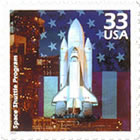#### You may also like### More Mods

What is the units digit for the number 123^(456) ?### Mod 3

Prove that if a^2+b^2 is a multiple of 3 then both a and b are multiples of 3.### Novemberish

a) A four digit number (in base 10) aabb is a perfect square. Discuss ways of systematically finding this number. (b) Prove that 11^{10}-1 is divisible by 100.

# Check Code Sensitivity

##### Age 14 to 16Challenge LevelCheck codes are designed to pick up common errors such as transposing two digits or miscopying a single digit. A person types the code number into a machine which decides whether it is a valid code or not. If someone types in a US Postal Service Money Order number and makes a single error, just one mistake in one digit, will the machine pick up every error of this type? Will a machine always pick up an error in a single digit for an airline ticket number?

US Postal Service Money Order: This is an eleven digit number using digits 1,2,...9 where the sum of the first ten digits is congruent to the eleventh digit modulo 9. That is $a_1a_2\cdots a_{11}$ where $a_1+ \cdots +a_{10} \equiv a_{11}$ mod $9$.

Airline tickets: This number can be any length. It uses the digits 0 to 9 and the last digit is a check digit. The number formed by omitting the check digit must be congruent to the check digit modulo 7.
That is $a_1\cdots a_na_{n+1}$ where $a_1a_2\cdots a_n \equiv a_{n+1}$ mod 7.